# How to use the MUNIT Function in Excel

A unit or identity matrix is matrix having all diagonals values starting from the uppermost left value to the lowermost right value.

A unit or identity matrix of 3 x 3 dimension is shown below.
This means the matrix has 3 rows and 3 columns.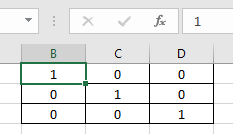A unit or identity matrix is a square matrix i.e. Square matrix is, which have an equal number of rows and columns.
Determinant of a unit or square matrix of any n x n dimension is 1.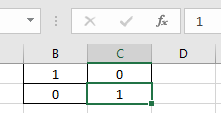According to the formula
| A | = 1 * 1 - 0 * 0
| A | = 1
The MUNIT function returns a unit matrix of n x n, where n is the dimension for the resulting matrix.
Syntax:

=MUNIT(dimension)

Dimension : input the n (number) which returns n x n matrix.

This is not easy to return output from the function.

Follow the steps to use the MUNIT function. Now I will show how the MUNIT function works to get us the unit matrix or identity matrix of 5 x 5 dimension

1. First select the 25 cells along the table of 5 x 5 dimension as shown below.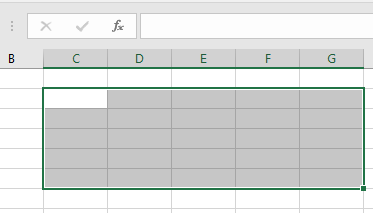1. Now
Use the formula:
{ = MUNIT ( 5 ) }

Note : don’t use the curly braces using the keyboard symbol. Use the Ctrl + Shift + Enter to apply the curly braces as shown below.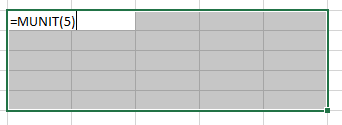1. Now press the Ctrl + Shift + Enter to apply the formula on the selected cell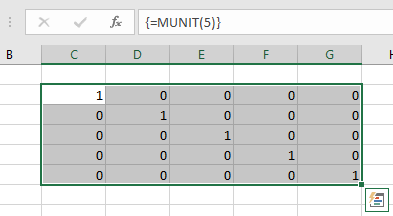Here is it. As you can see here the MUNIT function returns the unit matrix of 5 x 5 following the steps as explained.

Notes:

1. The function returns the #VALUE! error if the value of dimension is less than or equal to 0.
2. The function returns the #NAME? Error is the value of dimension in non - numeric.
3. The function will not result the unit or identity matrix if the selected cell doesn’t have an equal number of rows & columns.
4. If you are using Office 365, you wouldn’t have to use the above procedure. You just have to type the formula in one cell =MUNIT(5) and press Enter to have the unit matrix or identity matrix 5 x 5 dimension.

Hope you understood how to use MUNIT function and referring cell in Excel. Explore more articles on Excel mathematical Matrix functions here. Please feel free to state your query or feedback for the above article.

Popular Articles:

50 Excel Shortcuts to Increase Your Productivity

How to use the VLOOKUP Function in Excel

How to use the COUNTIF in Excel 2016

How to use the SUMIF Function in Excel

Terms and Conditions of use

The applications/code on this site are distributed as is and without warranties or liability. In no event shall the owner of the copyrights, or the authors of the applications/code be liable for any loss of profit, any problems or any damage resulting from the use or evaluation of the applications/code.